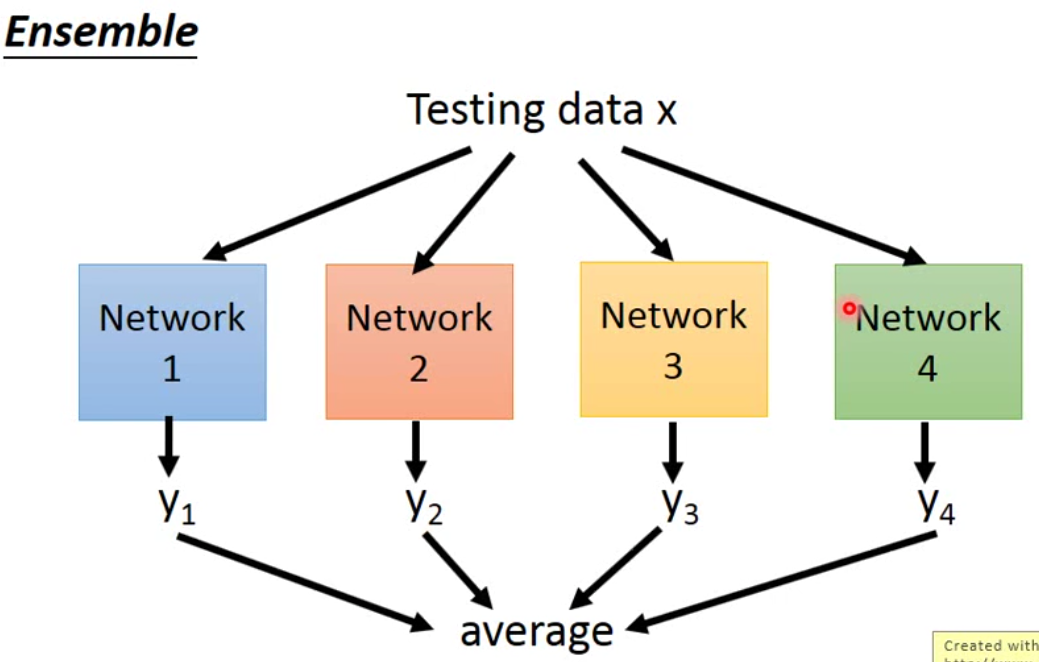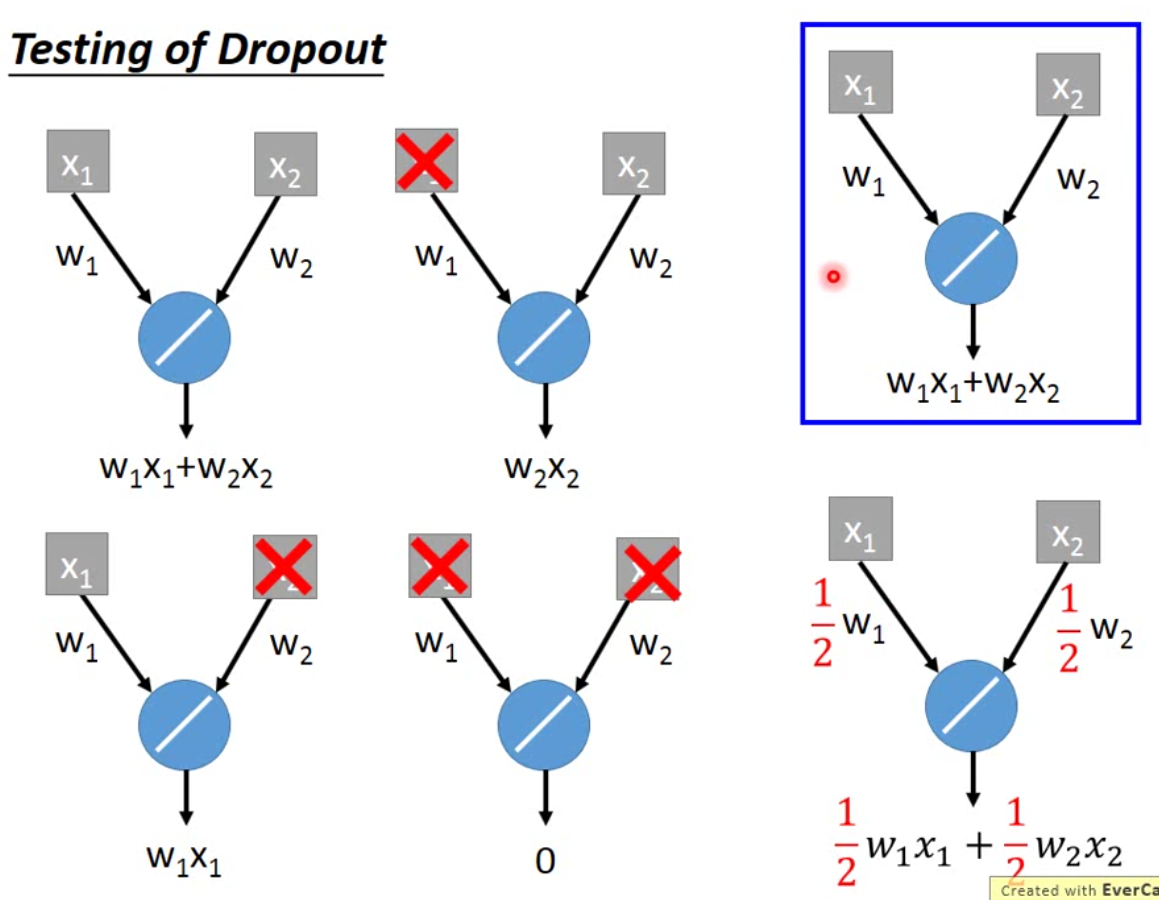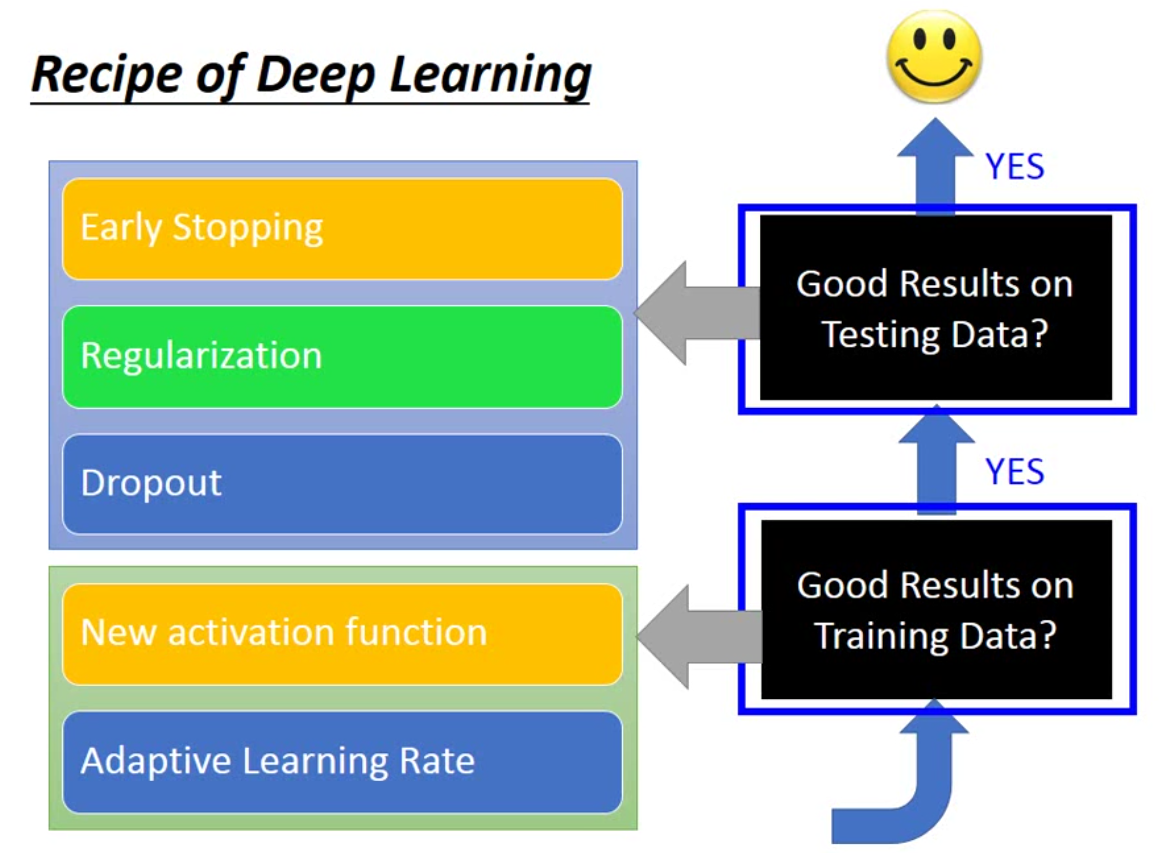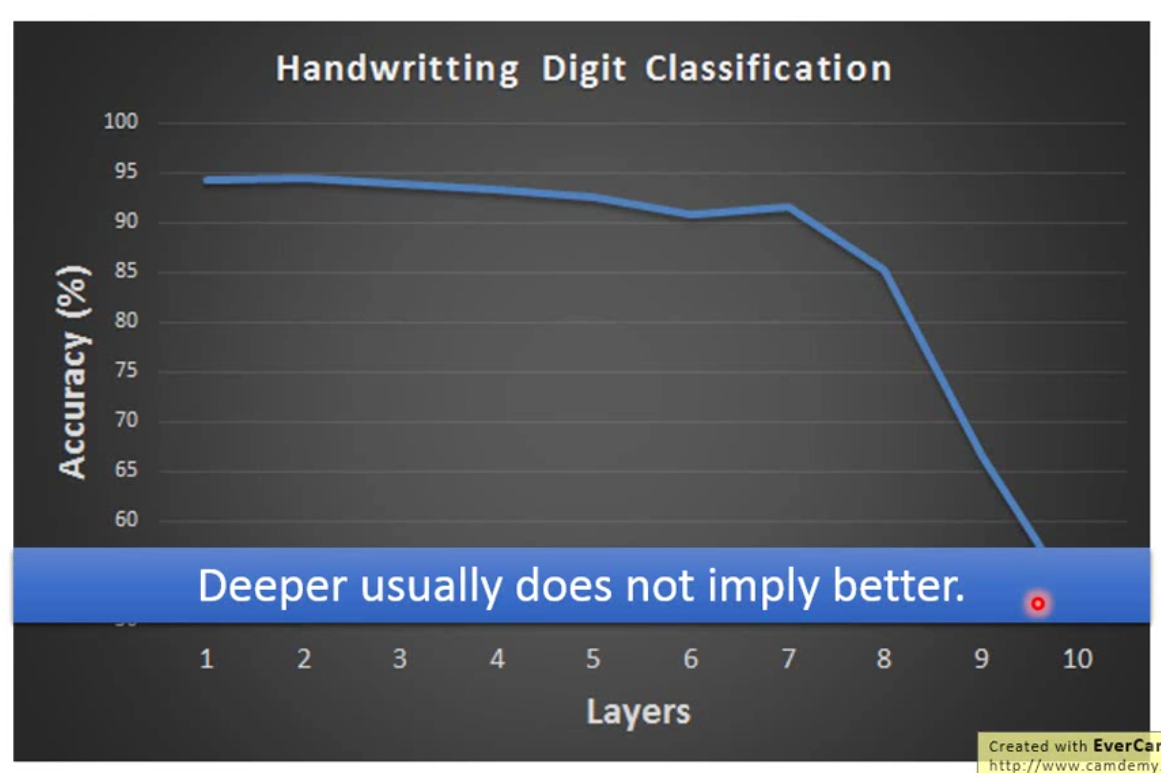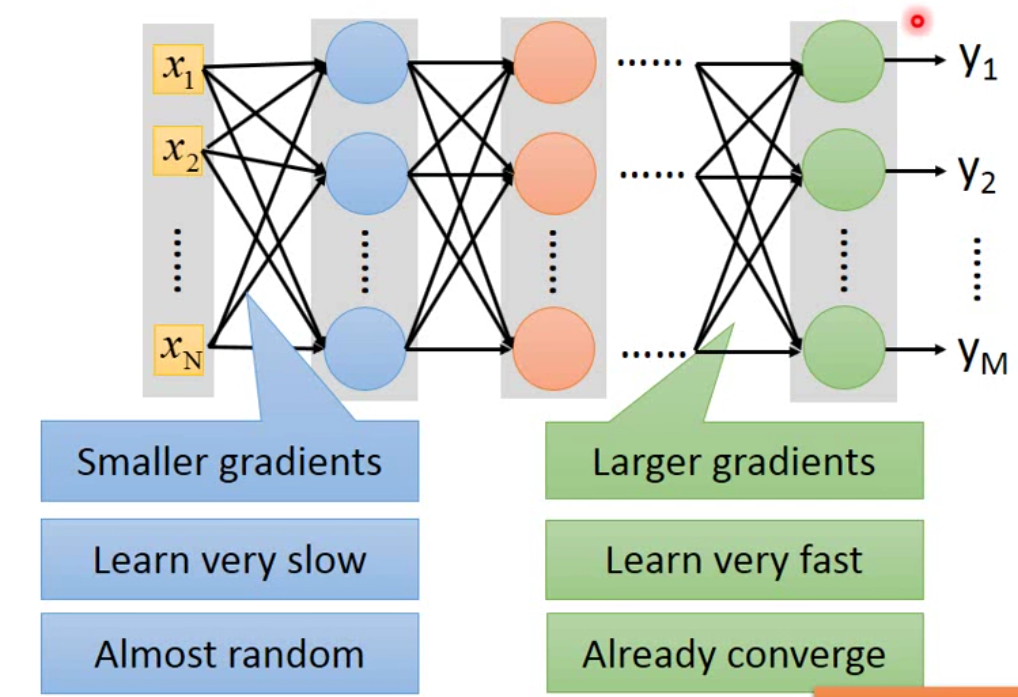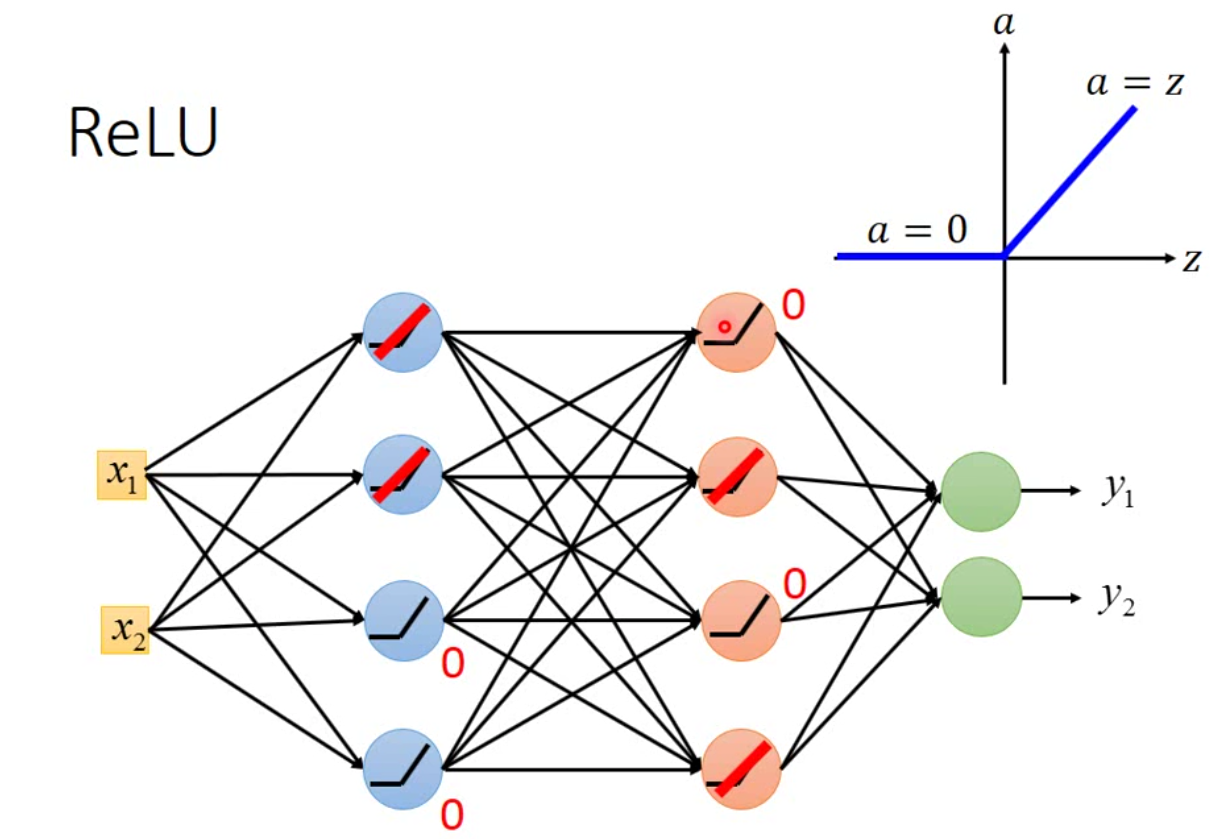ReLu有各种各样的变型，一种是Leaky ReLu： $a = \begin{cases} 0.01z, &\mbox{if }z<0 \\ z, &\mbox{if }z>0 \end{cases}$ 还有一个种是parametric ReLu： $a = \begin{cases} \alpha z, &\mbox{if }z<0 \\ z, &\mbox{if }z>0 \end{cases}$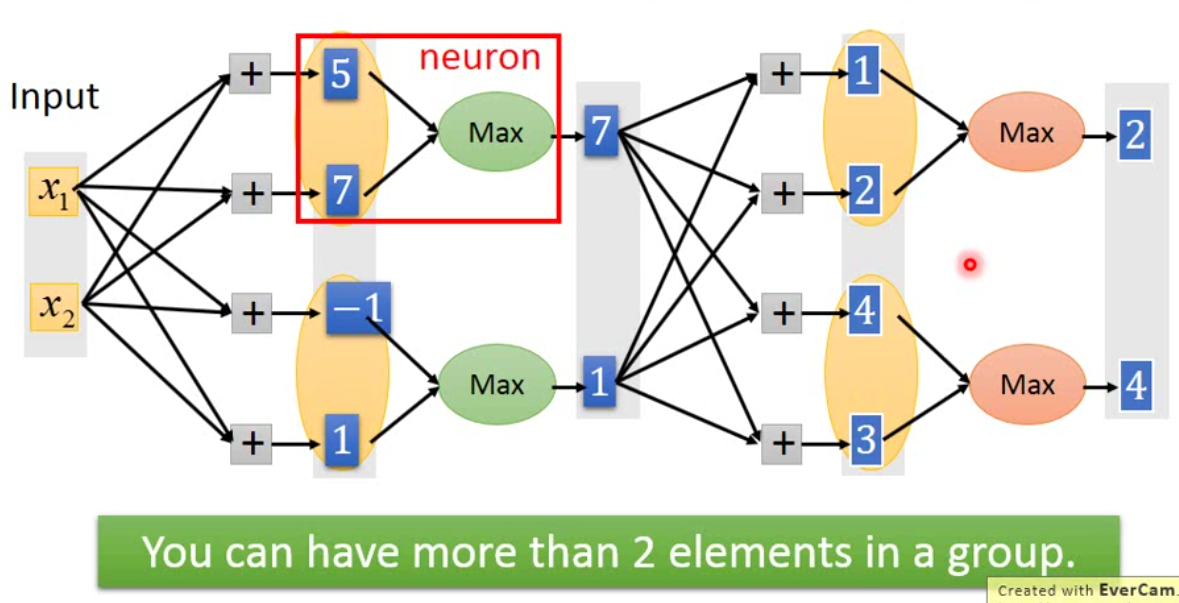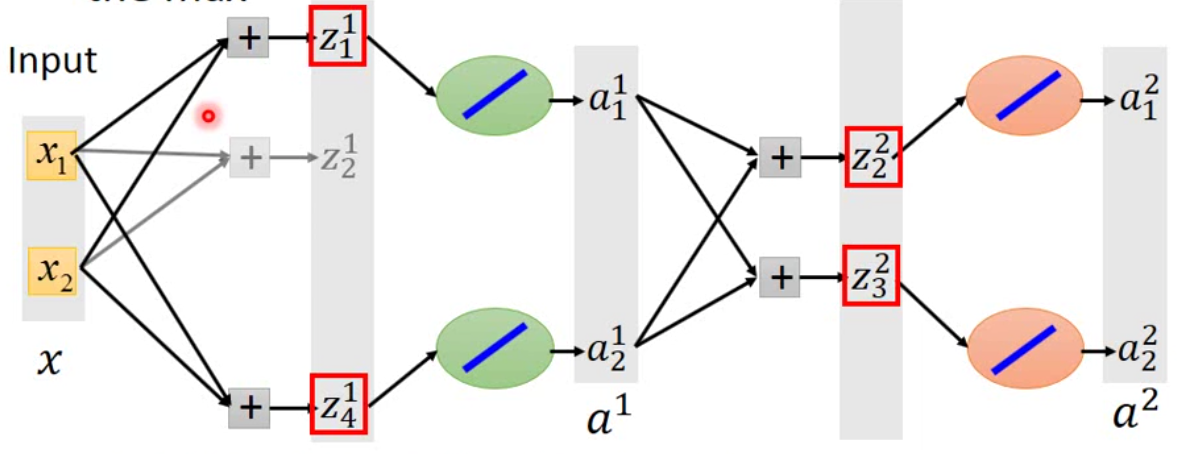Hinton 提出了一种新的更新方法，RMSProp，更新步骤如下： $w^{t+1} = w^t - \frac{\eta}{\sigma^t} g^t, \sigma^t = \sqrt{\alpha (\sigma^{t-1})^2 + (1-\alpha) (g^t)^2}$ 这是对Adagrad的一种变形。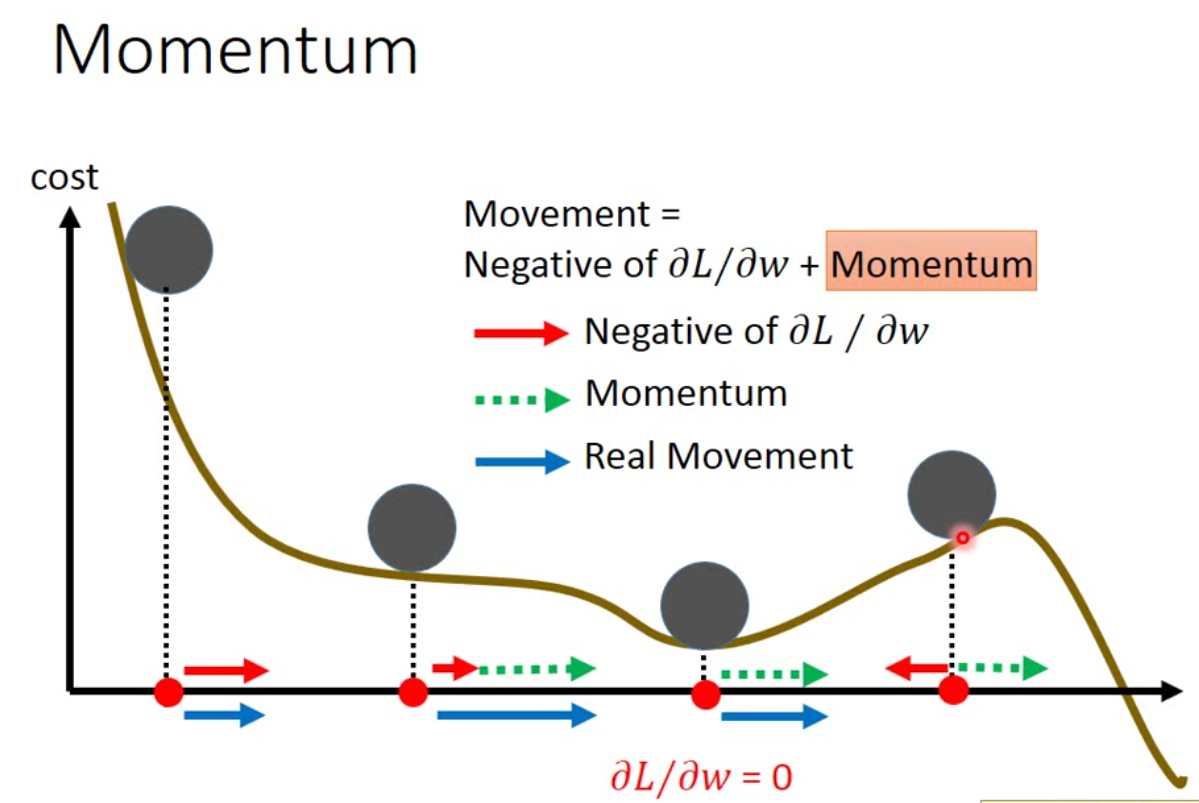L2 regularization表示如下： $L'(\theta) = L(\theta) + \lambda \frac{1}{2}||\theta||_2, \quad ||\theta||_2 = (w_1)^2 + (w_2)^2 + \dots + (w_n)^2$ 那么我们更新参数的方法就是： $w^{t+1} = w^t - \eta \frac{\partial L'}{\partial w} = w^t - \eta (\frac{\partial L}{\partial w} + \lambda w^t) = (1-\eta \lambda)w^t - \eta \frac{\partial L}{\partial w}$ 由于后面还跟了一个当前的梯度，因此不用担心这样的更新方法会导致所有的参数迭代到0。